# Polar zonohedra

Steven Dutch, Professor Emeritus, Natural and Applied Sciences, University of Wisconsin - Green Bay

## Zones, Zone Axes and zonohedra

A zone is a family of faces of a polyhedron that are all parallel to a given direction in space, called the zone axis. For example, the faces of a prism make up a zone whose zone axis is parallel to the axis of the prism. Only the orientation of the zone axis is important; it has no specific location. In the polyhedra below, the green and blue faces make up a zone with the zone axis vertical.In a trivial sense, every pair of faces on a polyhedron can be thought of as a zone, with the zone axis parallel to their mutual edge. But usually the term zone is applied only to groups of faces that make up a large part of a polyhedron, especially if the faces are related by symmetry.The solids above illustrate some other zone concepts. The four red faces on the cube at left obviously all belong to a zone, with the zone axis vertical. So do the four red octagon faces of the truncated cube to its right. If we continue truncating the cube, we get the cuboctahedron (third from left) which is halfway between a cube and an octahedron. The four red faces do not share edges, but they still constitute a zone with a vertical zone axis. So do the four red faces in the truncated octahedron (far right). So we can see that the faces in a zone need not intersect at all, as long as they are all parallel to the same line in space.

The truncated octahedron consists of squares and hexagons. The top green face and the hexagon below it intersect in a horizontal line, but the top hexagon intersects with the hexagon below it in a horizontal line also. That hexagon in turn intersects the bottom face in a horizontal line, and so on up the back of the polyhedron. We have a set of faces completely girdling the polyhedron, all parallel to a horizontal line. They make up a zone. Faces in a zone need not be identical and need not be limited to four sides.

We can see that if a face adjoins two neighboring faces of the same zone, it will have a pair of parallel edges. Solids where every edge is parallel to a zone axis are called zonohedra.

## Polar zonohedra

Polar zonohedra have a single principal symmetry axis. Below we see the 3- 4- and 5- fold polar zonohedra.Each zonohedron has n edges meeting at the top vertex. If these are the only edges allowed, then the faces around the vertex must be rhombuses. They in turn define the angles of the next face, which must also be a rhombus, and so on.

There is no rule regarding scale along the polar axis. The three-fold zonohedron is a rhombohedron, of which a cube is a special case. One special case of the four-fold zonohedron is the rhombic dodecahedron. But if the polyhedron is stretched a bit so the equatorial faces are squares, the top and bottom sets of faces are 60-120 rhombuses.

We could stretch the five-fold zonohedron a bit so that it is girdled by a zig-zag belt of squares. If we truncate the top and bottom pyramids, we are tempted to think we have a solid made of regular polygons. But alas, no - the triangles are not quite equilateral. But a cardboard model looks quite convincing and attractive.

We can see that each zonohedron has n faces around each vertex and n-1 bands of faces circling it, so a polar zonohedron has n(n-1) faces.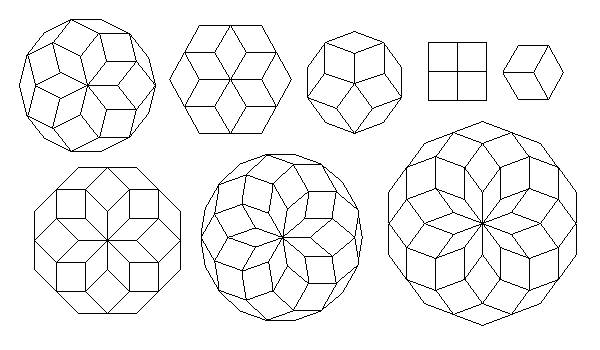Viewed along the polar axis (above), polar zonohedra form lovely rosettes. Each ring of rhombuses in turn dicates the next. If n is even, the limit of the polyhedron occurs when the angle between intersecting rhombus edges equals 180 degrees. The equatorial cross-section of the zonohedron has n sides. If n is odd, the limit of the polyhedron occurs when the angle between intersecting rhombus edges exceeds 180 degrees. The equatorial cross-section of the zonohedron has 2n sides.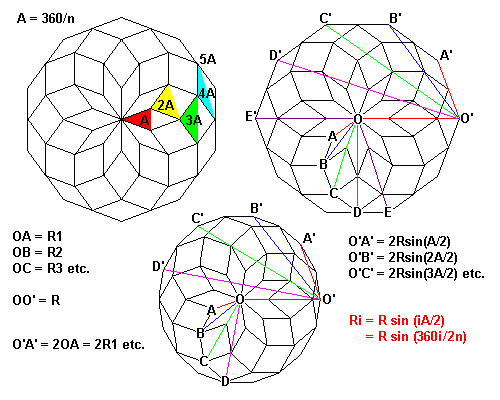Here we can see some basic geometry of zonohedra. At top left, we can see that the i-th ring of rhombuses has angle iA, where A = 360/n. This is true for any n. We'd also like to find a formula for the radius of each ring of vertices. Start numbering the rings with zero at the center. The formula is easiest to see for even n (top right). We can see that OE' is the diameter of a 2n-gon, and O'E' is the diameter of a 2n-gon twice as large. If we define R=O'E', then we have O'A'=2Rsin(A/2), O'B'=2Rsin(2A/2), O'C'=2Rsin(3A/2), and so on. If we define R1 = OA, R2 = OB, and so on, it's obvious that R1=O'A'/2, and so on. Therefore, Ri = Rsin(iA/2). When Ri = R, sin(iA/2)=1, iA/2=90=180i/n, and i=n/2.

It's a bit harder to see the geometry for n odd (bottom) because the edges in the rosette don't form replicas of the equatorial section, but the relationship is the same.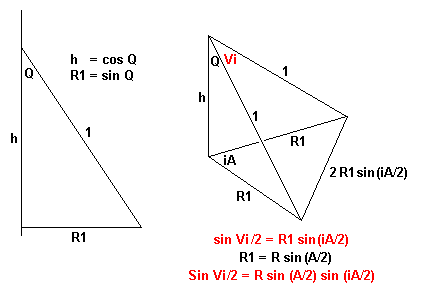Now let's look in the axial direction. If we define edge length as 1, and let the angle the edge makes with the zone axis = Q, then the projection along the axis of the edge is h. If we consider the edge at the top vertex, then the projection normal to the axis is R1.

Since all the edges are parallel to one of the edges at the top vertex:

• All edges have the same length projected on the polar axis
• All the vertices lie on equally spaced planes, and since
• Ri = Rsin(iA/2)
• The axial section of the zonohedron is a sine curve.
• As a fringe benefit, the formulas for vertex radius work for all values of i. When i = n, Ri=sin(nA/2)=sin(n 360/n /2)=sin(180) = 0, and we are at the bottom vertex. Also, for n odd, R(n-1)/2=R(n+1)/2. For example, when n=5, R2=R3.

The one final task is to get the face angles. We do this at right above. If Vi equals the vertex angle of a face in the i-th ring, then we find by straightforward trigonometry that SinVi/2=Rsin(A/2)sin(iA/2). At first glance, this looks strange. Where did angle Q go? Well, it turns out that angle Q determines R1, and hence R.

### In Terms of Edge Length = 1

• Length L of polar axis = nh = ncosQ
• Maximum radius R = sinQ/sin(A/2)
• SinVi/2 = sinQsin(iA/2)

### In Terms of Maximum Radius R

• Edge = Rsin(A/2)/sinQ
• Length L of polar axis = nh = ncosQ
• SinVi/2=Rsin(A/2)sin(iA/2)

### In Terms of Maximum Radius R and Polar Axis L

• CosQ = L/n; calculate SinQ
• Edge = Rsin(A/2)/sinQ
• SinVi/2=Rsin(A/2)sin(iA/2)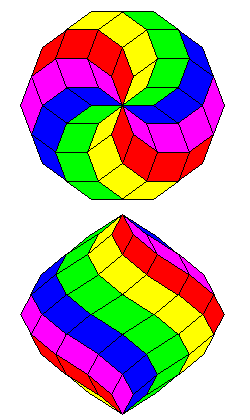Above is the 10-zonohedron, scaled so its polar axis and diameter are about equal. In this view the successive rings of rhombus faces are colored. Here the polyhedron is colored so that faces of a given zone have the same color.

### Some Special Cases

#### n=3

• Edge=1, A=120, A/2=60, sin(A/2)=sqrt(3)/2
• Sin(V1/2) = Rsin(A/2)sin(A/2) = 3R/4
• Sin(V2/2) = Rsin(A/2)sin(2A/2), but sin120 = sin 60 so V2=V1
• If V1=90 (a cube), Sin(V1/2)=sqrt(2)/2 and R=2sqrt(2)/3
• Since R = sinQ/sin(A/2), sinQ=Rsin(A/2)=Rsqrt(3)/2=sqrt(6)/3
• SinQ=sqrt(6)/3 implies CosQ=sqrt(3)/3
• L = 3cosQ = sqrt(3) - the correct length for a cube.

#### n=4

A=90, A/2=45, Sin(A/2)=sqrt(2)/2

• Case 1: V2=90.
• SinV2/2=Rsin(A/2)sin(2A/2)=Rsqrt(2)/2
• sqrt(2)/2=Rsqrt(2)/2, hence R=1
• SinV1/2=Rsin(A/2)sin(A/2)=R/2=1/2
• Thus V1/2=30 and V1=60
• Thus this solid has square equatorial faces and 60-120 top and bottom faces, as described earlier.

• Case 2: isohedral faces. V1=V2 does not work, so solve for V1=180-V2
• SinV1/2=Rsin(A/2)sin(A/2)=R/2
• SinV2/2=Rsin(A/2)sin(2A/2)=Rsqrt(2)/2=sqrt(2)sin(V1/2)
• Sin(V1/2)=sin((180-V2)/2)=sin(90-V2/2)=cos(V2/2)
• SinV2/2=sqrt(2)sin(V1/2), so sin2(v2/2)=2sin2(v1/2)
• cos2(v2/2)=1-2sin2(v1/2)=sin2(v1/2)
• Thus sin2(v1/2)=1/3, and sin(v1/2)=sqrt(3)/3
• V1/2=35.26, thus V1=70.53, the angles for a rhombic dodecahedron.

## Extended zonohedra

Let's try the method above for n=5, to see if there are any isohedral zonohedra.

A=72, A/2=36, Sin(A/2)=0.5877....

For simplicity, let sin36=v and sin72=w

• Again solve for V1=180-V2
• SinV1/2=Rsin(A/2)sin(A/2)=Rv2
• SinV2/2=Rsin(A/2)sin(2A/2)=Rvw=(w/v)SinV1/2
• Sin(V1/2)=sin((180-V2)/2)=sin(90-V2/2)=cos(V2/2)
• SinV2/2=(w/v)sin(V1/2), so sin2(v2/2)=(w/v)2sin2(v1/2)
• cos2(v2/2)=1-(w/v)2sin2(v1/2)=sin2(v1/2)
• Thus sin2(v1/2)=1/(1+(w/v)2), and sin(v1/2)=0.5257
• V1/2=31.72, thus V1=63.43, the angles for a rhombic triacontahedron.

So far, so good. Now let's calculate R and L

• Sin(V1/2) = sinQsin(A/2), so sinQ=Sin(V1/2)sin(A/2)=.8944
• Q = 63.43 degrees also. The vertex faces don't have a very steep slope.
• Cos Q = 0.4772
• L = 5cosQ = 2.236 = sqrt(5)
• R = sinQ/sin(A/2) = 0..8944/sin(36) = 1.5217

The diameter, 2R = 3.0434, but the length along the polar axis is only 2.236. We expect a rhombic triacontahedron to be roughly equidimensional. Wassup?

The solution is correct. It turns out there are several polyhedra that can be constructed with the same face as the rhombic triacontahedron. The solid we have actually constructed looks like this:Recall that we discovered that a polar zonohedron has n(n-1) faces. For n=5, there are 20 faces. There is a rosette of five faces around each vertex (red, yellow, orange and green) and a zigzag band around the middle (purple)

We can extend a zonohedron by adding a zone. We can separate the polyhedron along any set of edges, pull the two pieces apart, and join the two halves with equal and parallel edges.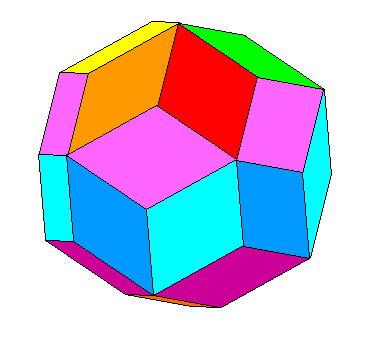The rhombic traicontahedron is actually made by extending the basic polyhedron above. We separate the zigzag band of purple faces, pull the two halves apart parallel to the polar axis by one edge length, and add a new set of edges. We add a girdle of ten faces around the equator. Note that these are identical to all the other faces.  Note that this particular extension will only work if n is odd.

## Diminished zonohedra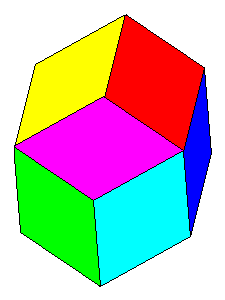We can also diminish a zonohedron by removing any zone. We can get a third polyhedron with rhombic triacontahedron faces by removing a zone from the 20-face zonohedron above. It looks like this and has 12 faces, but different symmetry from the regular rhombic dodecahedron. These three shapes, the rhombic dodecahedron, and the general rhombohedron are the only convex solids with congruent rhombic faces.

Created 19 January 2001, Last Update 11 June 2020